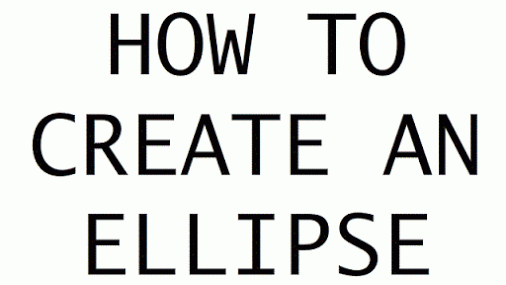# Section 15.4: Conic Sections

## 15.4 Outline

1. Introduction
1. definition
2. model
2. Geometric definition of conic sections
1. parabola
1. focus
2. directrix
3. axis
4. vertex
2. ellipse
1. foci
2. major axis
3. center
4. minor axis
3. circle
1. center
4. hyperbola
1. transverse axis
2. center
3. conjugate axis
3. Algebraic definition of conic sections
1. general form
1. first-degree equation
2. second-degree equation
2. line
3. parabola
4. ellipse
5. circle
6. hyperbola
4. Graphing conic sections
1. standard form
2. ellipses
1. standard-form equations
2. equation of a circle
3. horizontal ellipse
4. vertical ellipse
5. eccentricity
1. aphelion
2. perihelion
6. applications
3. hyperbolas
1. standard-form equations
2. vertices
3. horizontal hyperbola
4. vertical hyperbola
5. length of axis
6. applications
5. Parabolic reflectors

## 15.4 Essential Ideas

Geometric definition of the conic sections:

A parabola is the set of all points in the plane equidistant from a given point (called the focus) and a given line (called the directrix).

An ellipse is the set of all points in a plane such that, for each point on the ellipse, the sum of its distances from two fixed points (called the foci) is a constant.A circle (a special type of an ellipse) is the set of all points in a plane a given distance from a given point.

A hyperbola is the set of all points in a plane such that, for each point on the hyperbola, the difference of its distances from two fixed points (the foci) is a constant.

Algebraic definition of the conic sections:

The general form of the equation of a conic section is

Ax2 + Bxy + Cy2 + Dx + Ey + F = 0

where A, B, C, D, E, and F are real numbers and (x, y) is any point on the curve.

This equation is called a: first-degree equation if A = B = C =0
second-degree equation otherwise.

If B = 0, then we classify the conic section as follows: A = C = 0,
then the conic section is a line;

A = 0 and C is not equal to 0 or if A is not equal to 0 and C = 0, it is a parabola; A and C have the same sign, it is an ellipse;

A = C is a circle; and A and C have opposite signs, it is a hyperbola.

If B is not equal to 0, then the conic is rotated.